# Comparing spectrums: Sunlight and Earth’s surface emissions

Some people are apparently suspicious of the diagrams comparing the spectrum of the Sun and the spectrum of thermal emissions from Earth’s surface. There seems to be a belief that such diagrams are constructed in a deceptive way which conceals how much longwave radiation is actually present in sunlight. In this post, I’ll sort out these issues.

The bottom line is: no, nobody is deceptively claiming sunlight has less longwave radiation than it does. People who think that seem to be mistakenly ignoring the fact that the “inverse square law” exists, and that light gets less intense as it travels the 150 million kilometers from the Sun to the Earth.

At the same time, it is true that some diagrams comparing the of the spectrums of sunlight and Earth’s emissions are designed in ways that are a little bit “off.” I’ll explain the problems that some diagram designer run into, and I will offer a diagram that is avoids those problems.

## Sunlight at the surface of the Sun vs. at Earth

The Sun radiates much like a blackbody at a temperature of 5772 Kelvin. In contrast, the Earth’s surface radiates much like a blackbody with a temperature of around 288 K.1This is an approximation, since the temperature of Earth’s surface varies by location and time, and because the emissivity[LINK] is somewhat less than 1. However, the purpose of this sort of diagram is to offer an approximate illustration of the spectrum, and for that purpose, it’s reasonable to look at the emission spectrum of a blackbody at 228 K. The spectrum of radiation emitted by a blackbody at a given temperature is given by Planck’s Law. So, it’s easy to plot these two spectrums.2It’s considered acceptable to use either “spectrums” or “spectra” as the plural of “spectrum.”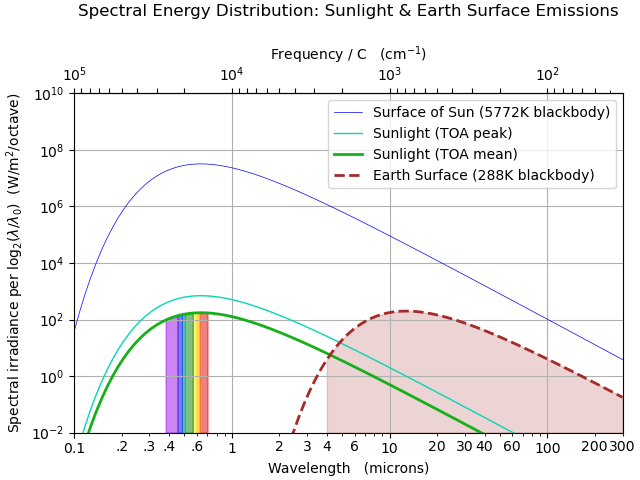Figure 1. Spectrum of sunlight (taken to be a blackbody at 5772K) and thermal radiation from Earth’s surface (taken to be a blackbody at 288K). Shadings show the colors of the visible spectrum, and the region considered to be “longwave radiation,” i.e., radiation with a wavelength longer than 4 microns. (How high the rainbow shading extends in this figure is not significant.)

In Figure 1, above, the blue top curve represents the spectrum of sunlight as it is emitted at the surface of the Sun, while the dark red dashed curve represents thermal emissions from the surface of the Earth. Comparing these curves, the Sun emits more radiation than Earth at all wavelengths.

However, the Sun’s radiation gets weaker as it travels the 150 million kilometers it must traverse to reach Earth. At the surface of the Sun, the total intensity of sunlight is 63,000,000 W/m2. However, by the time sunlight gets to Earth, the intensity is reduced to 1361 W/m2. (This is the intensity associated with the curve labelled as “TOA peak.”) That’s a reduction by a factor of 2.17×10-5 or 1/46200. Why is the intensity reduced so much? It’s the “inverse-square law.” Light spreading out in all directions from a localized source gets less intense as the inverse square of the distance traversed. The effective radius of the Sun is 696,340 km, while the distance to Earth is 149,600,000 km, so the intensity of radiation is reduced by a factor of (696340/149600000)2 = 2.17×10-5.

The spectrum of the solar peak radiation at the top of Earth’s atmosphere is shown by the teal curve in Figure 1. This curve represents the spectrum of sunlight at its peak intensity, as experienced above the top-of-the-atmosphere in full sunlight, facing in the direction of the Sun.

When comparing this curve to the spectrum of radiation emitted by Earth’s surface, it is no longer true that sunlight includes a “large” amount of longwave radiation. For wavelengths over 10 microns, sunlight is less than 1 percent as as intense as what is emitted by Earth’s surface.

And, less than 1 percent of the total power in sunlight is in the longwave region (i.e., has wavelengths longer than 4 microns).

When doing comparisons, it is arguably fairest to consider the mean intensity of sunlight at the top of the atmosphere. That mean (or average) is 1/4 of the peak value. This reflects the facts that (a) sunlight is present only half the time, while Earth emits thermal radiation 24 hours a day; and (b) sunlight is less intense when the Sun is not straight overhead.

The green curve in Figure 1 shows the mean spectrum of solar radiation inbound at the top of Earth’s atmosphere.

The curves relevant to radiation at Earth are shown below with a linear Y-axis.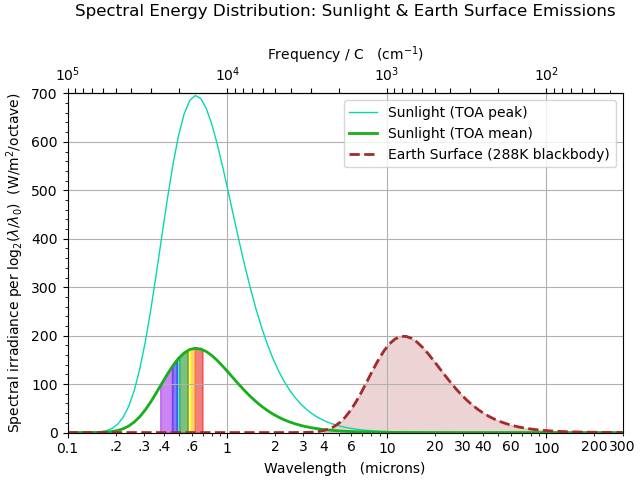Figure 2. Spectrum of inbound sunlight at the top of Earth’s atmosphere (TOA) and spectrum of thermal radiation from Earth’s surface. “TOA peak” sunlight corresponds to the value experienced during the daytime when the Sun is straight overhead, while “TOA mean” reflects sunlight averaged over day and night and over different locations.

Regardless of which version of the spectrum for sunlight one considers, it is evident that sunlight reaching Earth contains very little longwave radiation in comparison to the amount of longwave radiation emitted by Earth’s surface.

## Simple figure comparing spectrum of sunlight with emissions from Earth’s surface

Figure 3, below, offers what I consider to be a fair comparison of the spectrum of sunlight (before it enter’s Earth’s atmosphere), compared with the spectrum of thermal radiation emitted by Earth’s surface.

In the diagram, the area under each curve represents the amount of power associated with that type of radiation. So, for example, the area of the green part of of the rainbow shading represents the power of the green light in sunlight, while the red shaded region represents the power of the red light in sunlight.

The area under the the green curve to the left of the violet vertical band reflects the power of the ultraviolet radiation in sunlight. The area under the green curve to the right of the red vertical band reflects the power of the infrared radiation in sunlight. This infrared radiation is almost entirely shortwave infrared (with a wavelength shorter than 4 microns). Less than 1% of sunlight has a wavelength longer than 4 microns.

The total area under the green curve represents the total mean power of solar radiation reaching Earth, or about 340 W/m2. The area under the dashed red curve reflects the total emissions of a 288K blackbody, or 390 W/m2. (To anyone who believes the latter couldn’t possibly be larger than the former, I suggest reading my essay Energy Conservation allows Power to Appear seemingly from Nowhere.)

## Why some illustrations comparing spectrums are a little “off”

Note that there are no arbitrary normalization changes in the charts that I am offering. This is in contrast with sources that sometimes simply reduce the intensity of the solar spectrum by an arbitrary amount, rather than computing the exact reduction that is appropriate due the the inverse-square law.

It’s unfortunate but understandable that some people do that. Planck’s Law can be expressed in a number of ways. The more familiar ways of expressing Planck’s Law are not really designed to make intuitive sense when plotted using a logarithmic X-axis. Yet, using a logarithmic X-axis is the best way to offer a fair comparison between the spectrum of sunlight and the spectrum of Earth’s thermal emissions. So, people sometimes struggle to figure out how to plot the spectra with a logarithmic X-axis in a way that looks right and makes sense.

Below is plot of how the spectra look with a linear X-axis, using the wavelength version of Planck’s Law.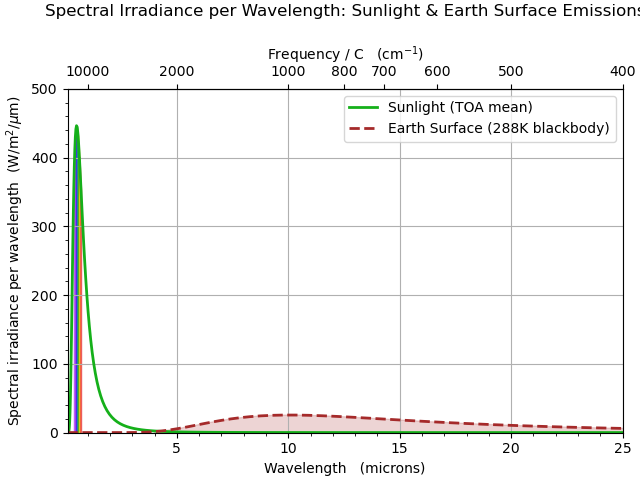Figure 4. Spectrums of inbound sunlight and thermal radiation from Earth’s surface, using wavelength version of Planck’s Law, plotted with a linear X-axis.

And, below is a plot of how the spectra look with a linear X-axis, using the frequency version of Planck’s Law.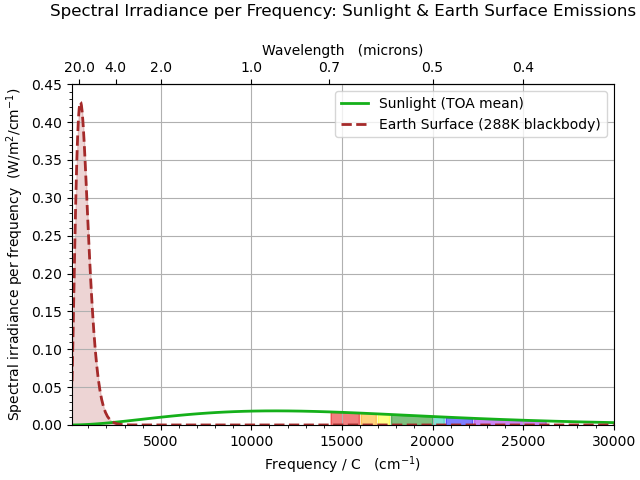Figure 5. Spectrums of inbound sunlight and thermal radiation from Earth’s surface, using frequency version of Planck’s Law, plotted with a linear X-axis.

These plots are both correct, but they make it difficult to compare the two spectrums. Also, notice that in the wavelength version, the peak of the solar spectrum is higher than the peak of the Earth surface emission spectrum, but in the frequency version, the relative heights are the other way around.

This observation becomes even more notable when these quantities are plotted with a logarithmic X-axis, as shown below.Figure 6. Log X-axis plot of frequency version of Planck spectra for sunlight and Earth’s surface emissions.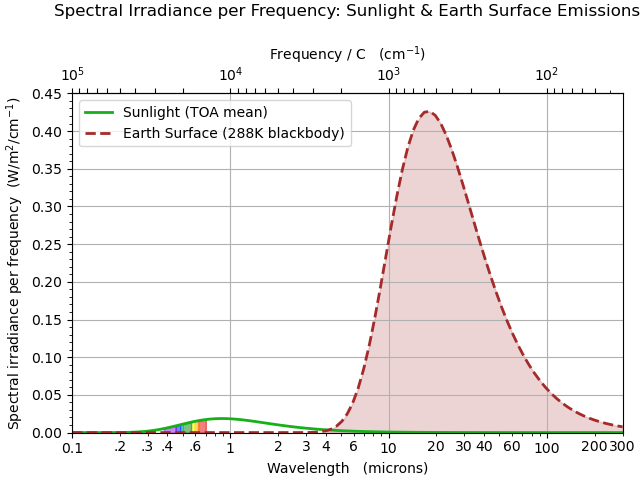Figure 7. Log X-axis plot of frequency version of Planck spectra for sunlight and Earth’s surface emissions.

I’ve made the X-axis identical in Figures 6 and 7, to support easy comparison. Yet, the spectra for sunlight and Earth’s surface emissions look very different in the two plots. The relative heights of the two spectra are very different. The locations of the peaks (or maxima) of the curves are different as well (which is why Wien’s Displacement Law has different versions, which yield different results, depending on whether one is working with spectrum with respect to wavelength or the spectrum with respect to frequency). Notice that the peaks of the curves are further to the right in Figure 7 than in Figure 6.

How can that be? Why do the spectra look so different, depending on whether one is plotting using the wavelength or frequency version of Planck’s Law?

It’s as if Figures 6 and 7 are plotting entirely different quantities! In fact, that’s true. The quantities are quite different, even if they’re related to one another.

Most people don’t realize that a “spectrum” is a complex and subtle mathematical object which isn’t nearly as simple as most people might assume. The spectrum isn’t simply “the” spectrum, it’s the spectrum with respect to a particular variable. The spectrum with respect to wavelength is not the same thing as the spectrum with respect to frequency. (And, it’s possible to compute the spectrum with respect to additional variables.)

The spectrum with respect to wavelength is designed to make sense when plotted using a linear wavelength scale, as in Figure 4. In Figure 4, the area under each curve corresponds to the total power associated with that type of radiation. It’s not designed to make sense when plotted using a logarithmic wavelength scale, as in Figure 5. In Figure 5, the area under the curves means nothing, and the height of the curves also means nothing that is intuitively meaningful.

Similarly, the spectrum with respect to frequency is designed to make sense when plotted using a linear frequency scale, as in Figure 5. It’s not designed to make sense when plotted with a logarithmic scale, as in Figure 7.

Unfortunately, some people simply “adjust” things by using the wavelength version or the frequency version, even though they are using a logarithmic X-axis, and applying an arbitrary scaling factor to make the two different spectra about the same size visually.

That’s not a proper procedure—although the results look vaguely like what one sees if one does things correctly, as in Figure 3. The results of that sort of “adjustment” aren’t “deceptive” in that the “adjusted” diagrams lead to conclusions pretty close to reality. Yet, one can do better.

## The right way of plotting a spectrum with a logarithmic X-axis

Plotting the spectrum of radiation is a bit like creating a flat map to show the surface of a spherical planet. There isn’t any unique “right way” of doing it. Each way of doing it has different properties, and is appropriate for different purposes.

In the case of a spectrum, the important issue is: On what sort of plot does the area under the curves correspond to the total power of the radiation?

If one is going to plot a spectrum using a logarithmic X-axis, it is best and clearest to do so in a way that leads to “area corresponds to power” on a plot with a logarithmic X-axis.

To achieve that, one needs to use a version of Planck’s Law that is with respect to the logarithm of wavelength (or with respect to the logarithm of frequency). That’s what I’ve done for Figures 1, 2 and 3. This form of Planck’s Law is less well-known than the wavelength and frequency versions. However, this form is widely used in astronomy, where such plots are referred to as plots of the Spectral Energy Distribution

(One can obtain the formula for the Spectral Energy Distribution, as I’ve plotted it, by starting with the wavelength version of Planck’s Law and multiplying it by 𝜆⋅log(2) where 𝜆 is wavelength, or by starting with the frequency version of Planck’s Law and multiplying it by 𝜈⋅log(2) where 𝜈 is frequency. The factor of log(2) means that the height of the spectrum relates to the power associated with each doubling of wavelength or frequency; others might choose a different normalization for this sort of spectrum.)

I hope this has helped clarify the proper comparison of the spectrum of sunlight with the spectrum of thermal radiation emitted by Earth’s surface.

## Addendum: Comparison including spectral ranges

Below is a variant of Figure 3 that clearly lays out the divisions between different regions of the electromagnetic spectrum.